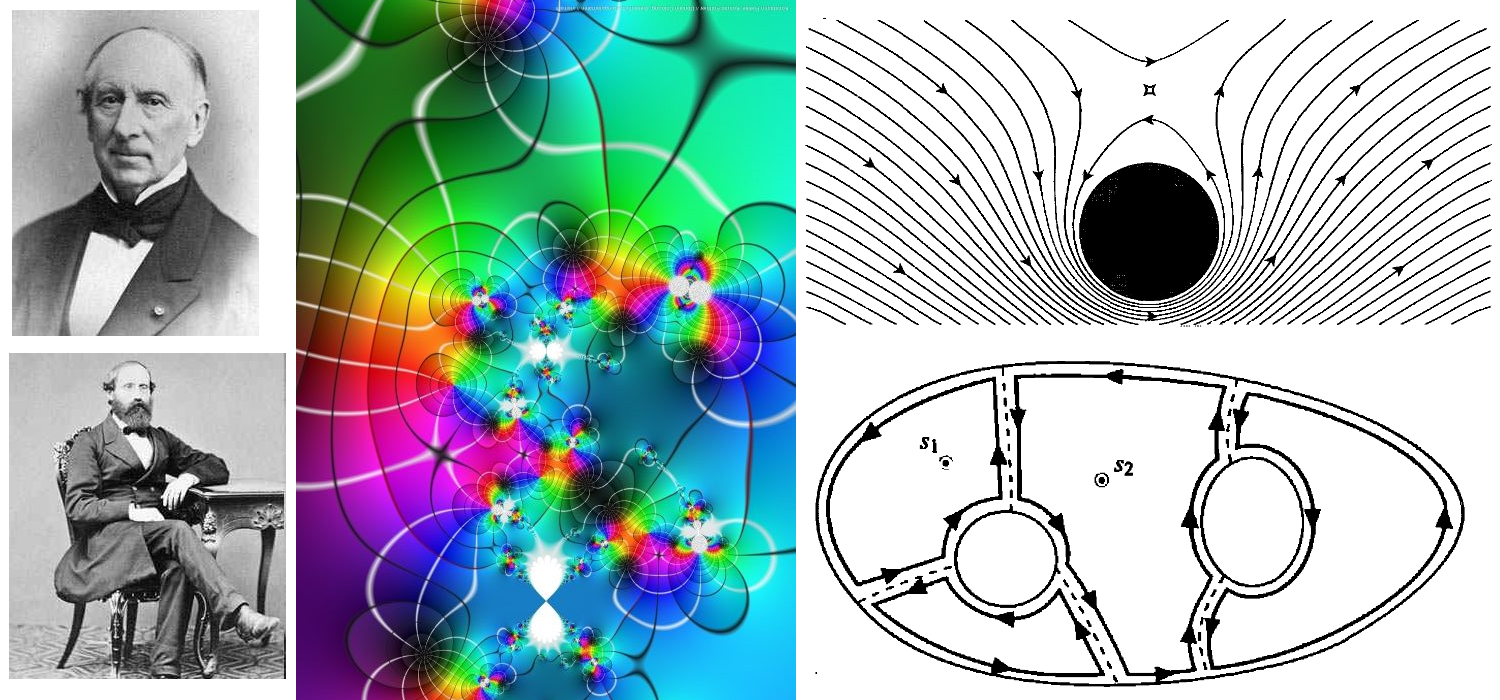#### Sets and Number Systems

To fully understand mathematics, the essential rules of logic for the formulation of mathematical proofs have to be studied. The various types of mathematical proofs are discussed, mainly using examples in elementary set theory and number systems.

In this course, students are introduced to the basic operations that are performed on sets. In so doing the concepts of relations, functions and binary operations are examined. Next we examine natural numbers and the derived principle of mathematical induction. We discuss permutations, combinations and sequences that are defined recursively with respect to natural numbers. The properties of the integers such as divisibility, greatest common divisor and Euclidean algorithm are examined. We study the field axioms in relation to rational numbers. We solve linear and non-linear inequalities involving the real numbers.#### Real Analysis 1

This course exposes students to rigorous mathematical definitions of limits of sequences of numbers and functions, classical results about continuity and series of numbers and their proofs. A major emphasis is placed on the proper use of definitions for the rigorous proof of theorems. The following topics will be covered: The real number system, topological properties of real numbers, sequences, continuity and uniform continuity.#### Complex Analysis

This course is an introduction to complex analysis, or the theory of the analytic functions of a complex variable. Such functions, beautiful on their own, are immediately useful in Physics, Engineering, and Signal Processing. However, the concepts introduced in complex analysis are nowadays also ubiquitous in number theory and combinatorics.

Students will calculate line integrals, and the techniques they will learn in this course will help them get past many of the seeming “dead ends” they ran up against in calculus. Indeed, most of the definite integrals they will learn to evaluate cannot be solved except through techniques from complex analysis.#### Topics in Numerical Analysis

Numerical analysis is the study of the computational methods used to solve problems involving continuous variables. It uses the ability of modern computers to perform billions of arithmetic operations per second, to find approximate solutions to otherwise intractable problems.

For example, students will survey some of the basic problems and methods needed to simulate the solutions of ordinary differential equations. Making sure that such a simulation is accurate but also can be performed fast is one of the main tasks in numerical analysis, e.g., when trying to predict the path of a tropical storm.

In this course, students will write programs to solve equations, approximate functions, integrate functions, and solve initial value problems. Thus this course will concentrate on the analytical side of numerical analysis, leaving direct and iterative methods in linear algebra to the course Linear Algebra 2.